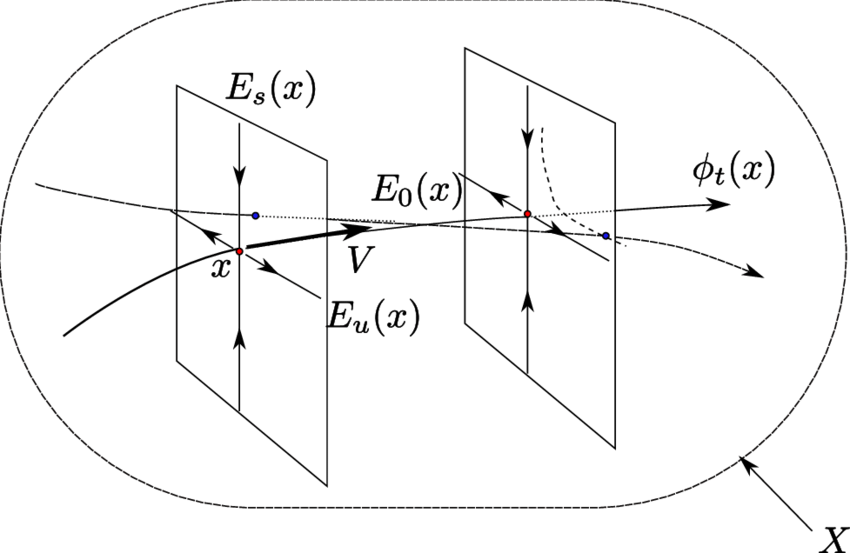# Rational factors, invariant foliations and algebraic disintegration of compact mixing Anosov flows of dimension 3An Anosov flow of dimension three.

Abstract: In this article, we develop a geometric framework to study the notion of semi-minimality for the generic type of a smooth autonomous differential equation $$(X,v)$$ , based on the study of rational factors of $$(X,v)$$ and of algebraic foliations on $$X$$ , invariant under the Lie-derivative of the vector field $$v$$.

We then illustrate the effectiveness of these methods by showing that certain autonomous algebraic differential equation of order three defined over the field of real numbers — more precisely, those associated to mixing, compact, Anosov flows of dimension three — are generically disintegrated.

Categories:

Updated: